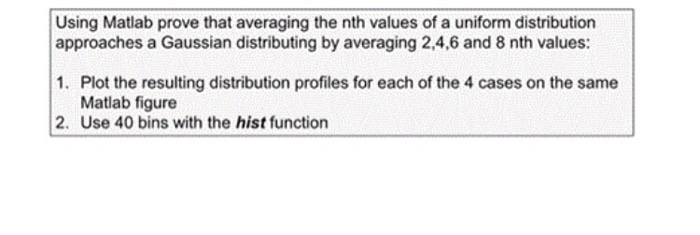# Homework Solution: Using Matlab prove that averaging the nth values of a uniform distribution approaches a Gaussian distribu…Using Matlab prove that averaging the nth values of a uniform distribution approaches a Gaussian distributing by averaging 2, 4, 6 and 8 nth values: 1. Plot the resulting distribution profiles for each of the 4 cases on the same Matlab figure 2. Use 40 bins with the hist function